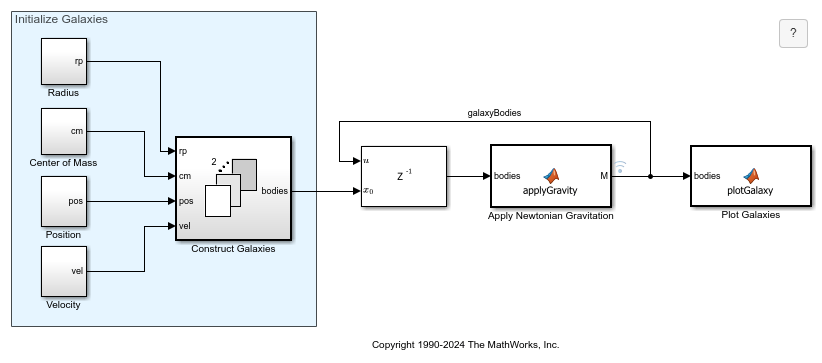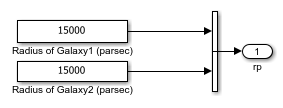Spiral Galaxy Formation Simulation Using MATLAB Function Blocks

This model was inspired by the classic paper "Galactic Bridges and Tails" (Toomre & Toomre 1972). The original paper explained how disc shaped galaxies could develop spiral arms. Two disc shape galaxies originally are far apart. They then fly by each other and almost collide. Once the galaxies are close enough, mutual gravitational forces cause spiral arms to form.

Except for the "PlotAll" visualization block's use of plotting routines, all MATLAB® function blocks in this model support code generation with Simulink® Coder™ and Embedded Coder™.

• Requirements: For this example, Simulink® generates code for the simulation in a Simulink project directory created in the current working directory (pwd). If you do not want to affect the current directory (or if you cannot generate files in this directory), you should change your working directory.

Opening the Model and Running the Simulation

Open the model and run the simulation by executing the code below. If you are using MATLAB® Help, select the code you want to execute and press F9 (or select code, right click on selection and select "Evaluate Selection"). The Simulink model will load, compile, and run.

model = 'sldemo_eml_galaxy';
open_system(model);
sim(model);Model Description

This section describes the model in detail and explains the role of each block in the model. The green blocks initialize the simulation, the orange blocks are the core of the simulation, and the yellow block makes the galaxy animation view.

Initial Conditions

The model requires initial conditions for each galaxy. The initial conditions are: galaxy radius in parsecs (rp), galaxy mass in solar mass units (cm), galaxy position in parsecs (pos), and galaxy velocity in m/s (vel).

In the model, constant blocks specify the initial conditions. The initial conditions have been chosen such that the galaxies will nearly collide at some point in time.

"ConstructGalaxy" Blocks

The initial conditions are passed to the MATLAB function blocks Construct Galaxy 1 and Construct Galaxy 2. These MATLAB function blocks contain MATLAB code that builds the galaxy models.

In a typical galaxy, most of the mass is concentrated in its center as a super-massive black hole and/or star agglomeration. We model the galaxy as a disc with radius r with most of its mass concentrated in the inner circle of radius r/3. In addition to this super-massive nucleus, the "ConstructGalaxy" MATLAB function block creates 349 random stars with masses ranging from 4 to 24 solar masses. These stars are randomly positioned within distance r/3 and r from the center of the galaxy. The stars initially move in circular orbits around the galaxy core. Every object (star or galaxy core) has mass, position (x, y, z), and velocity (Vx, Vy, Vz).

"Matrix Concatenation" Block

This block joins information about both galaxies. At this point the model has 700 objects: 1 core for each galaxy and 349 stars around each core. These 700 objects interact according to Newtonian mechanics.

"Partition" Block

This MATLAB function block separates all 700 objects into two groups: heavy bodies and light bodies. The heavy bodies are the galaxy cores. The light bodies are the stars. Because the galaxy cores are much heavier than individual stars, the model will consider only the heavy-heavy and heavy-light interactions. We can ignore the light-light body interactions. This will save a lot of time since 698 out of 700 bodies in the model are light.

"ApplyGravity" Block

This MATLAB function block uses Newtonian mechanics to compute the velocities and positions of the bodies at each step. The "combine" block is also a MATLAB function block. It merges the data about heavy and light objects together.

"PlotAll" Block

This MATLAB function block plots the bodies in a figure and updates the position of each star at every step in the simulation.

Closing the Model

Close the model without saving any changes. Clear data generated by simulation/example.

close_system(model,0);
clear model sldemo_eml_galaxy_output;

Note:

• In this model, signal logging is turned on (observe that the 'GalaxyBodies' signal has a little blue signal logging antenna on it). The model saves output data in a Dataset object. Any other data is saved/modified in the model workspace to avoid cluttering the MATLAB workspace.

• To modify signal logging settings, right-click on the signal line and select "Signal Properties". In this example, the signal name is 'GalaxyBodies' and the "Log signal data" checkbox is checked.

• The logged data is saved to the MATLAB workspace as a Dataset object with the name 'sldemo_eml_galaxy_output'. Information on the 'GalaxyBodies' signal can be retrieved from this object by typing sldemo_eml_galaxy_output.get('GalaxyBodies') which returns a Simulink.SimulationData.Signal object. See more about working with these objects in the "Simulink.SimulationData.Signal" documentation.